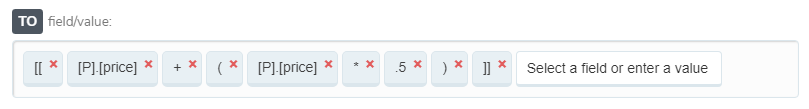# Feed rules: calculator functionGoDataFeed Technical Team
• Updated

Apply basic arithmetic to the numeric fields in your data feed with a custom rule.## Examples

Static Text: Orange, Fields: Blue

## Create a calculator function

1. Name rule
2. SET the feed field you would like to adjust
3. TO create a calculation
•  `[[`  Start the formula with two opening brackets
• Select your number field from your product catalog - fields starting with [P]
• Use any common operator in your formula, such as,
• Subtraction `-`
• Addition `+`
• Multiplication `*`
• Division `/`
• `]]` Close the formula with two closing brackets